# Substrate Integrated Waveguide

Substrate integrated waveguide (SIW) is an new form of transmission line that has been popularized in the past few years by some researchers. New transmission lines only come along once in a lifetime, so pay attention, this is a big deal!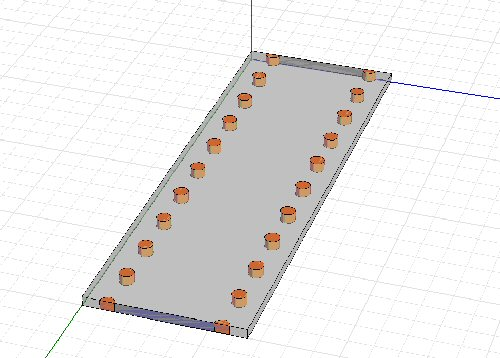SIW is shown in the HFSS model above. A rectangular guide is created within a substrate (usually a soft board) by adding a top metal over the ground plane and caging the structure with rows of plated vias on either side. To an EM wave, if everything works out, it looks like a dielectrically-filled rectangular waveguide, with reduced height compared to the "normal" 2:1 width:height ratio. Reduced height is no big deal, it just reduces the impedance the wave sees (increases capacitance/length).

The tradeoffs of any transmission media line start with its attenuation characteristics. Read our page on transmission line loss, to become familiar with the four loss mechanisms αC, αD , αG and αR.

One attraction to SIW is that the amount of metal that carries the signal is far greater than it would be in microstrip or stripline. Therefore conductor loss αC is lower.

One potential disadvantage if SIW is that leakage losses can be substantial. This is related to how tight the vias are spaced. This means that αR is a non-zero term.

Another disadvantage is that by introducing a dielectric into the guide (compared to air in "normal" rectangular waveguide) you now have introduced dielectric losses αD . This term is proportional to frequency, so the application of SIW at millimeter-wave needs to look at this term carefully. Chances are that losses due to conductivity of the substrate αG will be close to zero if you chose a good substrate. If you try this trick on high-resistivity silicon, let us know how much you lose due to conduction!

Because it is a waveguide, SIW exhibits a lower cut-off frequency.

Update November 2010! This new material came from Sinan, a Masters student at Bilkent University in Ankara, Turkey. Thank you, sir!

### Substrate Integrated Waveguide (SIW)

#### 1. Introduction

In high frequency applications, microstrip devices are not efficient, and because wavelength at high frequencies are small, microstrip device manufacturing requires very tight tolerances. At high frequencies waveguide devices are preferred; however their manufacturing process is difficult. Therefore a new concept emerged: substrate integrated waveguide. SIW is a transition between microstrip and dielectric-filled waveguide (DFW). Dielectric filled waveguide is converted to substrate integrated waveguide (SIW) by the help of vias for the side walls of the waveguide.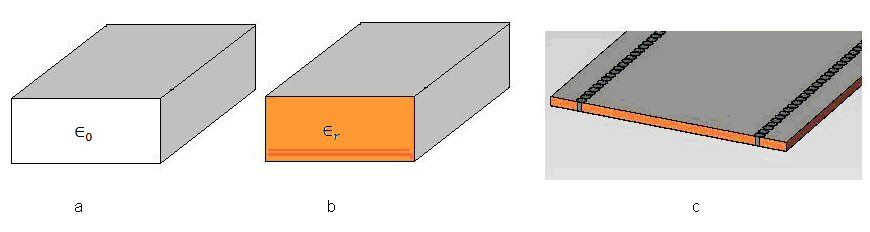Figure 1: (a) Air filled waveguide, (b) dielectric filled waveguide, (c) substrate integrated waveguide

Because there are vias at the sidewalls, transverse magnetic (TM) modes do not exist; TE10 therefore is the dominant mode. There are many articles for designing substrate integrated waveguides, however there are missing parts in many of them, they just give the known equations but they do not carefully investigate those equations. In this report, the tolerances of the published SIW design equations are inspected.

#### 2. SIW Design Equations

SIW devices can be thought as a form of dielectric filled waveguide (DFW), therefore the starting point can be DFW. For TE10 mode, the dimension "b" is not important as it does not affect the cut off frequency of the waveguide. Therefore the substrate can be at any thickness; it only affects the dielectric loss (thicker=lower loss).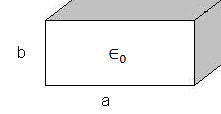Figure 2: Dimension definition of rectangular waveguide

For a rectangular waveguide, cut off frequency of arbitrary mode is found by the following formula: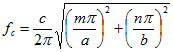(1)

where:
c: speed of light
m, n: mode numbers
a, b: dimensions of the waveguide

For TE10 mode, the much-simplified version of this formula is: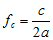(2)

For DFW with same cut off frequency, dimension "ad" is found by: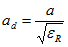(3)

Having determined the dimension "a" for the DFW, we can now pass to the design equations for SIW.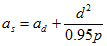(4)

where
d: diameter of the via
p: pitch (distance between the vias)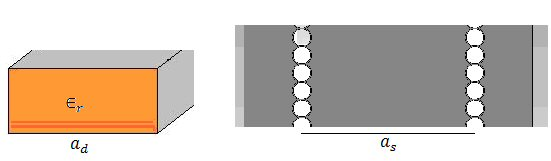Figure 3: Dimensions for DFW and SIW

In published articles about SIW design, the following two conditions are required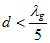(5)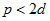(6)

Where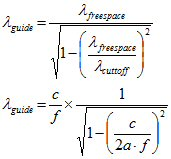(guided wavelength) is: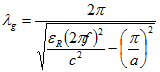(7)

#### 3. Investigation of the Equations

In this part, we investigated (5) and (6) equations and the geometry in Figure 4 is used for testing. The substrate is Rogers 3003, 10 mil with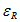=3.0. We designed a SIW at Ka band. As seen from Figure 5, pitch and diameter are measured from the centers.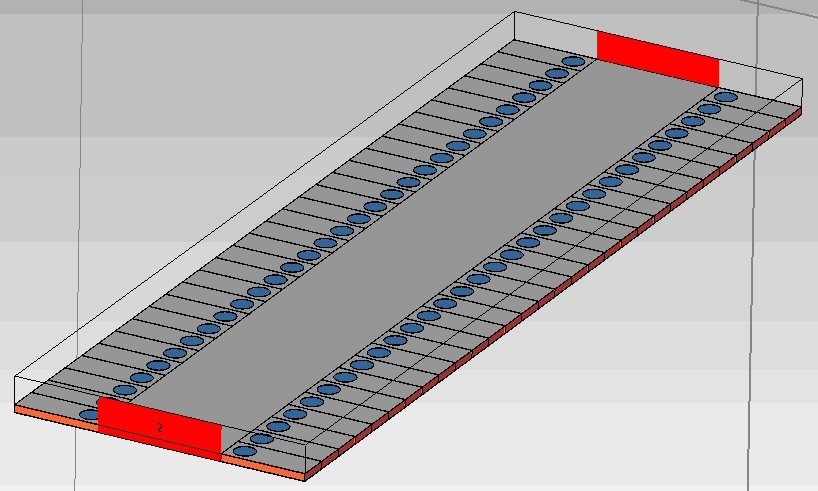Figure 4: Geometry of SIW for testing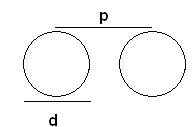Figure 5: Diameter and pitch

Ka band is used at 26.5-40 GHz and for the maximum case (40 GHz), we investigated the results. At 40 GHz,= 179 mil, therefore maximum d is 35.8 mils. Diameter sweep results of CST are shown in Figure 6 and pitch sweep results are in Figure 7.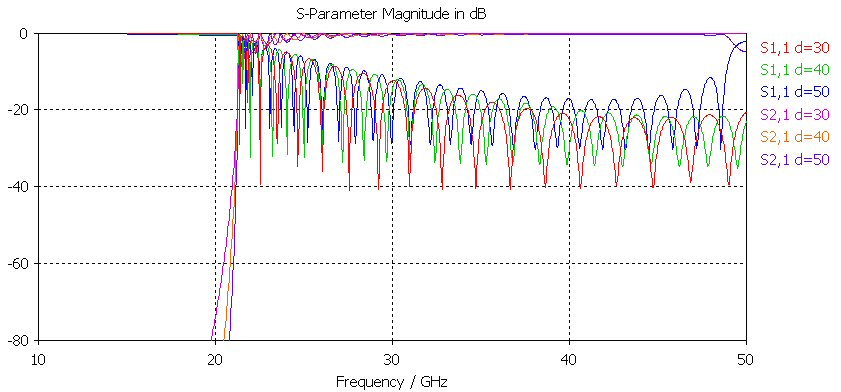Figure 6: Results for d=30, 40, 50 mil and p=1.5d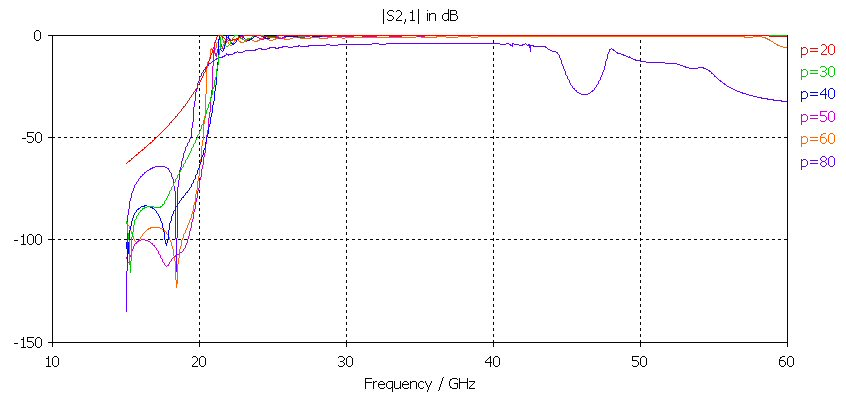Figure 7: Results for p=20, 30, 40, 50, 60, 80 and d=20 mil

#### 4. Conclusion

As seen from Figure 6, when the diameter increases, the bandwidth narrows from the higher frequency side. The result for d=50 mil can be inspected in this figure.

As seen from Figure 7, when the pitch increases, the bandwidth narrows from the higher frequency side and for higher values of p, the response is distorted. The responses for p=60 and 80 should be inspected.

When we investigated the results of Figures 6 and 7, it is clear that these design equations work well, however they are not obligatory for designing SIW. They can be used as initial design equations and after the first design they can be optimized if the frequency range to be used is different. These equations are necessary for finding the equivalent waveguide.

#### 5. References

 K. Wu, D. Deslandes and Y. Cassivi, "The Substrate Integrated Circuits - A New Concept for High-Frequency Electronics and Optoelectronics," TELSKIS 2003, Nis, Serbia and Montenegro, pp. Oct. 2003.

 J. E. Rayas-Sanchez and V. Gutierrez-Ayala, "A General EM-Based Design Procedure for Single-Layer Substrate Integrated Waveguide Interconnects with Microstrip Transitions", IEEE MTT-S Int. Microwave Symp. Dig., Atlanta, GA, Jun. 2008, pp. 983-986.

Author : Unknown Editor